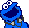###Author Topic: 7000 ID's  (Read 2023 times)

#### zombie.cxnt

We reached to now 7000 players since yesterday.

#### Slugger

I thought it would happen soon :D.

IDs in the 7000s != 7000 players

#### Bones4

Yay @ snots smartnesses. :D

Lol, Snot is the only person with his rank in his name

#### Dante

IDs in the 7000s != 7000 players

Not realy, -99 that dont get sold and -2 that are special numbers.

Warning - while you were reading a new reply has been posted. You may wish to review your post.

#### Anybody

We reached to now 7000 players since yesterday.#### zombie.cxnt

Not realy, -99 that dont get sold and -2 that are special numbers.

Warning - while you were reading a new reply has been posted. You may wish to review your post.

-X for members who bought more then one account can you figure out X?

Warning - while you were typing a new reply has been posted. You may wish to review your post.

Not realy, -99 that dont get sold and -2 that are special numbers.

Warning - while you were reading a new reply has been posted. You may wish to review your post.

"!=" means does not equal

#### Cogizio

Exactly snot. Exactly.

#### MegaScience

Code: [Select]
`OperatorsAssignment operators= Assigns the value of the second operand to the first operand.Mathematical Operators: + (Addition) Adds 2 numbers - (subtraction) Subtracts the value of its argument. * (Multiplication) Multiplies 2 numbers./ (Division) Divides 2 numbers.% (Modulus) Computes the integer remainder of dividing 2 numbers.+= Adds 2 numbers and assigns the result to the first.-= Subtracts 2 numbers and assigns the result to the first.*= Multiplies 2 numbers and assigns the result to the first./= Divides 2 numbers and assigns the result to the first.%= Computes the modulus of 2 numbers and assigns the result to the first.++ (Increment) Adds one to a variable representing a number (returning either the new or old value of the variable)-- (Decrement) Subtracts one from a variable representing a number (returning either the new or old value of the variable)Bitwise Operators: ~ (Bitwise NOT) Flips the bits of its operand.| (Bitwise OR) Returns a one in a bit if bits of either operand is one.& (Bitwise AND) Returns a one in each bit position if bits of both operands are ones.^ (Bitwise XOR) Returns a one in a bit position if bits of one but not both operands are one. << (Left shift) Shifts its first operand in binary representation the number of bits to the left specified in the second operand, shifting in zeros from the right.>> (Sign-propagating right shift) Shifts the first operand in binary representation the number of bits to the right specified in the second operand, discarding bits shifted off.|= Performs a bitwise OR and assigns the result to the first operand.&= Performs a bitwise AND and assigns the result to the first operand.^= Performs a bitwise XOR and assigns the result to the first operand.<<= Performs a left shift and assigns the result to the first operand.>>= Performs a sign-propagating right shift and assigns the result to the first operand.String operators:: @ Concatenates one or more values together to form a new valueNL Concatenates one value together with a new line to form a new valueTAB Concatenates one value together with a tab to form a new valueSPC Concatenates one value together with a space to form a new valueLogical Operators: ! evaluates the opposite of the value specified&& requires both values to be true for the result to be true.|| requires only one value to be true for the result to be true. Relational Operators: == value1 and value2 are equal!= value1 and value2 are not equal< value1 is less than value2> value1 is greater than value2<= value1 is less than or equal to value2>= value1 is greater than or equal to value2String comparison Operators: \$= string1 is equal to string2!\$= string1 is not equal to string2`Now do you understand?

#### yuki

In-Short:  "!=" is "Not Equal to"

#### Amiret

6025
thats around how many

#### Maxwell

When did we reach 7,000? I never even saw anyone in the 7k.

I saw 6958.